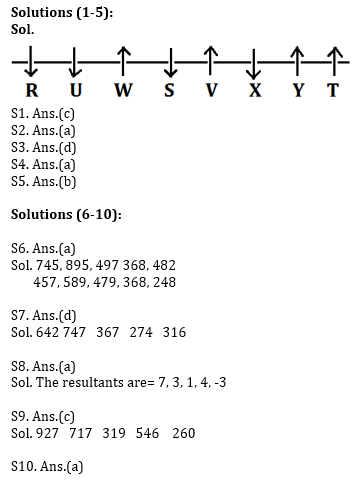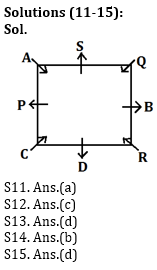# Reasoning Ability Quiz for Prelims Exams- SBI & IBPS 2020- 29th December

Directions (1-5): Study the following information carefully and answer the question given below.
Eight friends are sitting in a straight line. Some of them face north while some of them face south direction. R who sits one of the extreme end, sits third to the right of S. Y sits second to the right of V. Only three persons sit between W and Y who does not sit at extreme end of the row. V sits third to the left of U who is an immediate neighbour of W. V does not face south. T sits second to the left of X who sits third to the right of W. R sits left of T and facing opposite direction of T. Y faces north direction.

Q1. Who amongst the following sits exactly between W and Y?
(a) S
(b) R
(c) V
(d) X
(e) T

Q2. Who amongst the following sits third to the left of Y?
(a) S
(b) T
(c) U
(d) V
(e) W

Q3. Four of the following five are alike in a certain way and so form a group, which among the following does not belong to that group?
(a) R
(b) S
(c) X
(d) Y
(e) U

Q4. How many people sit between Y and U?
(a) Four
(b) Three
(c) None
(d) Two
(e) One

Q5. Who among the following sits fourth to the right of one who sits immediate right of V?
(a) W
(b) U
(c) X
(d) T
(e) Y

Directions (6-10): The following questions are based on the five three digits numbers given below:
634     784    386    257    371

Q6. If 1 is added in all the digits of each number and then digits are arranged in ascending order within the number, which of the following will be the second lowest number after rearrangement?
(a) 257
(b) 784
(c) 634
(d) 371
(e) None of these

Q7. If second digit is subtracted by one, then it is interchanged with third digit in each number, then which number will be the highest number after rearrangement?
(a) 257
(b) 371
(c) 386
(d) 784
(e) None of these

Q8. If in each number, both first and third digits are added and after that second digit is subtracted from that addition then how many numbers are greater than 5 after mentioned operation?
(a) One
(b) Two
(c) Three
(d) Four
(e) None of these

Q9. If in each number, we subtract 1 in all the odd digits and add 3 in all even digits then, which of the following will be the lowest number after mentioned operation?
(a) 634
(b) 386
(c) 371
(d) 784
(e) None of these

Q10. If all the digits in number are arranged in descending order, then which number is highest number?
(a) 784
(b) 634
(c) 257
(d) 386
(e) None of these

Directions (11-15): Study the following information carefully and answer the question given below:

Eight friends are sitting around a square table in such a way that four of them sit at four corners of the square while the other four sits in the middle of each sides. The ones who sit at the four corners face inside while those who sit in the middle of the sides face outside.
A sits third to the right of D. B sits second to the left of the one, who sits opposite to S. Q sits immediate left of the one, who sits immediate right of R. A sits opposite to R. P sits opposite to B. C does not sits at the middle of the side.

Q11. Who is sitting exactly between P and B, when counted right of P?
(a) S
(b) Q
(c) C
(d) A
(e) R

Q12. How many persons sit between D and Q when counted left of Q?
(a) Four
(b) Three
(c) Two
(d) One
(e) None

Q13. Who among the following sits immediate right of P?
(a) R
(b) C
(c) Q
(d) A
(e) B

Q14. Four of the following are alike in a certain way so form a group, which among the following does not belong to that group?
(a) S
(b) Q
(c) P
(d) D
(e) B

Q15. Who among the following sits third left of the one who sits second to the right of Q?
(a) S
(b) C
(c) R
(d) B
(e) P

Practice More Questions of Reasoning for Competitive Exams:

###### Prelims Quiz Study Plan for SBI & IBPS Exams 2020

SolutionsPractice with Crash Course and Online Test Series for IBPS Clerk Prelims:×

Thank You, Your details have been submitted we will get back to you.
×
OR

×
OR